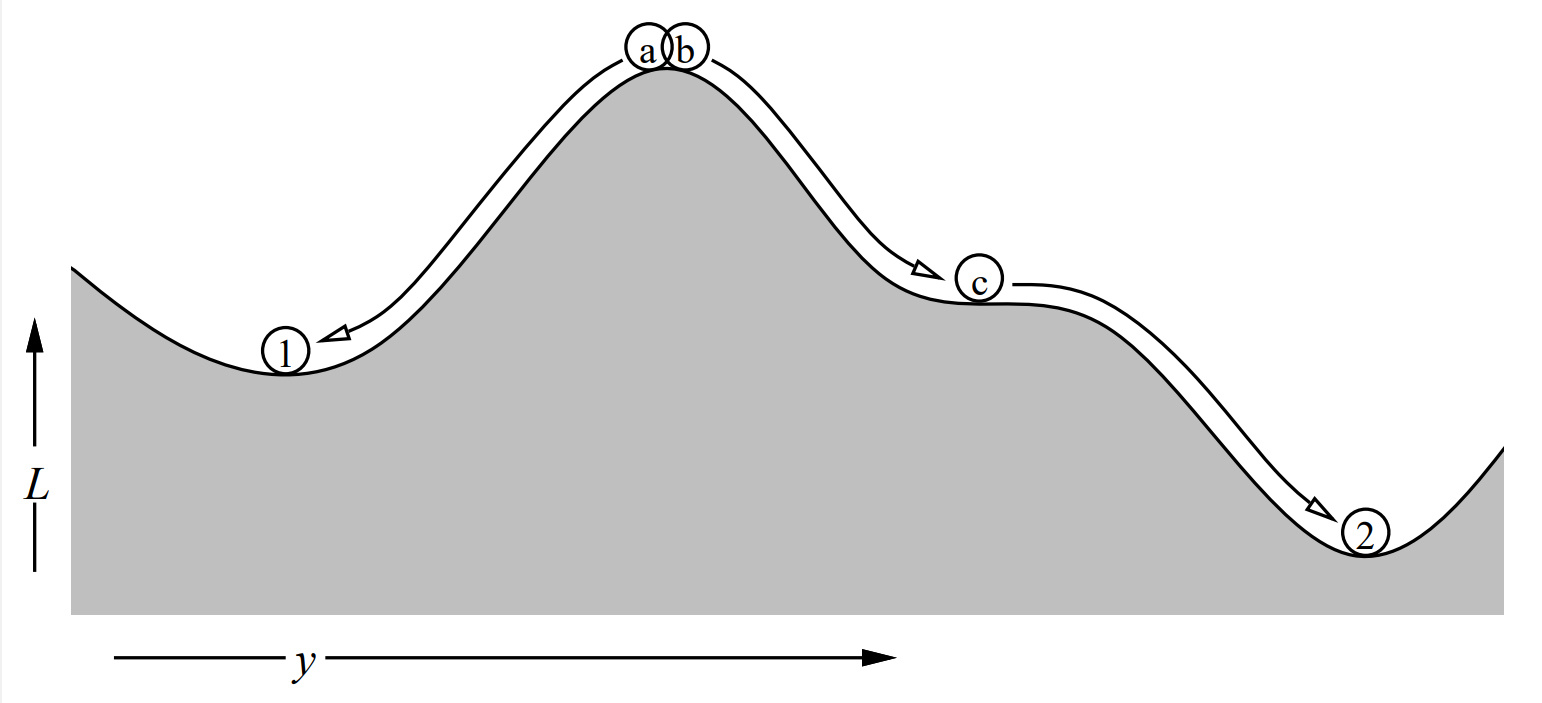# Backpropagation: Training Neural Networks

##### Technical Consultant

Backpropagation is so commonplace in neural network training that it is easy to take it for granted. But what is it?

Since its introduction in the 1980s, backpropagation has become a popular algorithm used to train neural network models and their hidden units. It uses a defined error/cost function and gradient descent to find a set of weights, iteratively, that optimizes the model for a particular task. Despite its simplicity, backpropagation has proven effective in training the neural networks needed for real-world applications (nonlinear networks with arbitrary connectivity).

### A Simple Illustration

Let us imagine a simple three-layer, fully connected, feedforward network consisting of an input layer (X), a hidden layer (H), and an output layer (Y). Let Xᵢ, Hⱼ, and Oₖ be individual nodes in the input, hidden, and output layers, respectively. So, the input layer has i nodes (X₁ to Xᵢ), the hidden layer has j nodes (H₁ to Hⱼ), and the output layer has k nodes (O₁ to Oₖ). The initial weights are random. These weights exist between the input layer-to-hidden layer (Wᵢₕ) and hidden layer-to-output layer (Wₕₒ). We also have a data set, D, to train the network. The dataset consists of l example inputs (S₁ to Sₗ) and their expected outputs (t₁ to tₗ).

The input layer encodes the data fed to it, one at a time, and then sends it to the hidden layer. The hidden layer takes that encoding, re-encodes it, and sends it to the output layer. Each hidden layer node (Hⱼ) takes in data from all input layer nodes weighted with their respective weights. For example, the output from node I₁ is weighted with W₁₁ and sent to the hidden layer node H₁. The hidden layer node runs all its inputs through its activation function, f(x). Additionally, a bias (β) is applied, and the result is the output of that hidden layer node.

Hₒᵤₜ = f(X₁, X₂, …, Xᵢ) + β

The output layer ingests the hidden layer encoding similarly. The output of the output layer is the output of the network. This process of the data “moving” from the input layer, through the hidden layer, to the output layer is called forward propagation. Naturally, backpropagation moves in the opposite direction.

Training a neural network model means adjusting its weights (e.g., Wᵢₕ and Wₕₒ) to minimize the model’s error. The error is the difference between the model’s output and the ground truth (e.g., tₗ). Training the model uses the sample data in the training set, and training repeats until the model demonstrates good performance. To see how training works for a single training input during a single training epoch (one complete pass through the training set), let us follow the journey of training input Sₗ.

Sₗ is run through the network, resulting in some output (Yₗ). Yₗ is compared to Dₗ’s associated ground truth (tₗ) using a pre-defined error function. Wₕₒ is adjusted to minimize the error between Yₗ and tₗ. tₗ is then “projected” onto the hidden layer using the adjusted Wₕₒ weights, producing a hidden layer encoding of Yₗ (Yₕ₁). Then, Wᵢₕ is adjusted similarly using Yₕ₁

### A Deeper Look

So, what exactly is backpropagation? Well, the formula is:

ln P(D|N) = Σ ln P(dᵢ|Sᵢ Λ D) + Σ ln P(Sᵢ)

The first term, Σ ln P(dₗ|Sᵢ Λ D), is the term to look at when training the network. It measures how well the model accounts for the data (i.e., the model’s performance). To find the optimal model, maximize this term. For a given value of Sₗ, there is a distribution of dₗ values that the network can predict. However, the model only predicts one value. So, we have to optimize the model for the target value. In other words, for a given value of Sₗ, the model should predict tₗ. So dₗ and tₗ should be the same.

The second term, Σ ln P(Sᵢ), is the prior probability or prior. It is the probability of Sₗ absent any relevant contextual information that may indicate Sₗ. We get priors from external sources (e.g., previous experiments or observations). They add information like a baseline probability or constraints to the model. They can also combat overfitting. Overfitting is when the model fits the training data but does not generalize well to new/novel input. In other words, instead of settling at a global minimum, the model gets stuck at a local minimum.Instead of reaching the global minimum (2) during training, the neural network can get stuck at a local minimum (1).Image credit Pearlmutter

Regularization can combat overfitting. One kind of regularization is weight decay. Weight decay assumes that the weights are normally distributed about a zero mean. Weight decay changes the weight adjustment process. Weights are now adjusted to reduce the overall error and to move the weights towards zero. The new process penalizes large weights, and the magnitude of the penalty varies according to chosen parameters.

Another kind of regularization is weight elimination. Weight elimination works on the assumption that simpler models fitting the data are better than complex ones. So, the weights are in a normal distribution centered at zero. So, small weights are adjusted conventionally, while large weights are changed little. Thus, small weights move towards zero and are eliminated, while large weights are left alone.

Banner image credit to
AndSus
Neural Networks